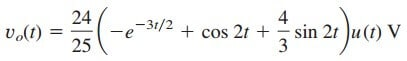# Laplace Transform Transfer Functions Examples and SolutionsWe have learned about the transfer functions back when we learn about the filter transfer function. Now we will implement the Laplace transform transfer function.

The transfer function is a key concept in signal processing because it indicates how a signal is processed as it passes through a network. It is a fitting tool for finding the network response, determining (or designing for) network stability, and network synthesis.

## Laplace Transform Transfer Functions

The transfer function of a network describes how the output behaves with respect to the input. It specifies the transfer from the input to the output in the s domain, assuming no initial energy.

The transfer function H(s) is the ratio of the output response Y(s) to the input excitation X(s), assuming all initial conditions are zero.

Thus

The transfer function H(s) is the ratio of the output response Y(s) to the input excitation X(s), assuming all initial conditions are zero.

Thus, a circuit can have many transfer functions. Note that H(s) is dimensionless in Equations.(2a) and (2b).

Each of the transfer functions in Equations.(2) can be found in two ways. One way is to assume any convenient input X ( s ), use any circuit analysis technique (such as current or voltage division, nodal or mesh analysis) to find the output Y (s ), and then obtain the ratio of the two.

The other approach is to apply the ladder method, which involves walking our way through the circuit. By this approach, we assume that the output is 1 V or 1 A as appropriate and use the basic laws of Ohm and Kirchhoff (KCL only) to obtain the input.

The transfer function becomes unity divided by the input. This approach may be more convenient to use when the circuit has many loops or nodes so that applying nodal or mesh analysis becomes cumbersome.

In the first method, we assume an input and find the output; in the second method, we assume the output and find the input. In both methods, we calculate H(s) as the ratio of output to input transforms.

The two methods rely on the linearity property since we only deal with linear circuits until now.

Equations.(1) assumes that both X(s) and Y(s) are known. Sometimes, we know the input X(s) and the transfer function H(s). We find the output Y (s ) as

and take the inverse transform to get y(t). A special case is when the
input is the unit impulse function, x(t) = δ(t), so that X(s) = 1. For this case,

where

The term h(t) represents the unit impulse response—it is the time-domain response of the network to a unit impulse. Thus, Equations.(5) provides a new interpretation for the transfer function: H(s) is the Laplace transform of the unit impulse response of the network.

Once we know the impulse response h(t) of a network, we can obtain the response of the network to any input signal using Equations.(5) in the s domain or using the convolution integral.

## Laplace Transform Transfer Functions Examples

1. The output of a linear system is
y(t) = 10et cos 4tu(t)
when the input is

x(t) = etu(t).
Find the transfer function of the system and its impulse
response.

Solution:
If x(t) = etu(t) and y(t) = 10etcos 4tu(t), then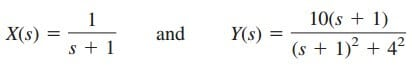Hence,To find h(t), we write H(s) asFrom the Table.(1) in the Laplace transform inverse,2. Determine the transfer function H(s) = Vo(s)/Io(s) of the circuit in Figure.(1)

Solution:
METHOD 1: By current division,But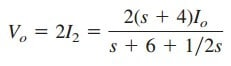Hence,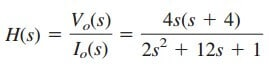METHOD 2: We can apply the ladder method. We let Vo = 1 V. By Ohm’s law, I2 = Vo/2 = 1/2 A.
The voltage across the (2 + 1/2s)
impedance is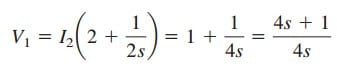This is the same as the voltage across the (s + 4) impedance. Hence,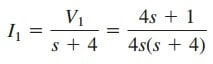Applying KCL at the top node yieldsHence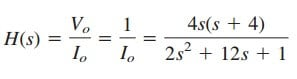as before.

3. For the s-domain circuit in Figure.(2), find:
(a) the transfer function H(s) = Vo/Vi ,
(b) the impulse response,
(c) the response when vi (t) = u(t) V,
(d) the response when vi (t) = 8 cos 2t V

Solution:
(a) Using voltage division,

But

Substituting Equations.(3.2) into (3.1) results in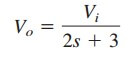Thus, the impulse response is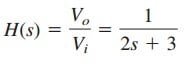(b) We may write H (s) asIts inverse Laplace transform is the required impulse response: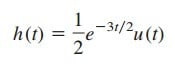(c) When vi (t) = u(t), Vi (s) = 1 /s, andwhere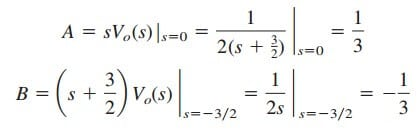Hence, for vi (t) = u(t),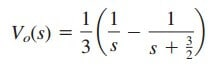and its inverse Laplace transform is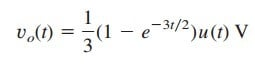(d) When vi(t) = 8 cos 2t, thenand

whereTo get B and C, we multiply Equation.(3.3) by (s + 3/2)(s2 + 4). We getEquating coefficients,Solving these gives A = −24/25, B = 24/25, C = 64/25.
Hence, for vi (t) = 8 cos 2t V,and its inverse is